## A posteriori error estimates for the Richards equation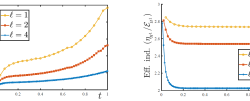Main results: nonlinear advection–reaction–diffusion equation; parabolic–hyperbolic and parabolic–elliptic degeneracies; a posteriori error estimates: guaranteed upper bound in nonlocal-in-time (time-integrated) norms; local space–time efficiency in standard norms; the reliability and efficiency norms coincide when there is no nonlinearity; distinction of error components. Details: preprint with Koondanibha Mitra

## Stable broken polynomial extensions and p-robust a posteriori error estimates in H(curl)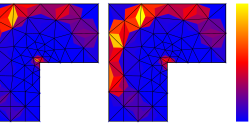Main results: H(curl)-stable polynomial extension on a tetrahedron; stable broken H(curl) polynomial extension on a patch of thetrahedra; a posteriori error estimates of H(curl)-conforming methods: reliable (guaranteed), locally efficient, polynomial-degree-robust, and inexpensive. Details: H(curl)-stable polynomial extension on a tetrahedron: paper (preprint) with Alexandre Ern and Théophile Chaumont-Frelet; stable broken polynomial…

## Robust a posteriori error estimates for reaction–diffusion problems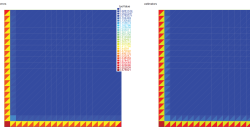Main results: steady linear reaction–diffusion equation; arbitrary-order finite element approximation, arbitrary space dimension; a posteriori error estimates: guaranteed upper bound in the energy norm; local efficiency; robustness with respect to reaction dominance; inexpensive construction (weights; no submesh, no combination with residual estimates); counter-example where estimates with no weights/submesh/combination fail; explicit…

## Robust a posteriori error estimates for linear advection problems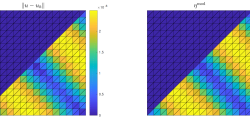Main results: steady linear advection equation; rigorous results in 1D, heuristic extensions to mD; a posteriori error estimates: guaranteed upper bound in the L2 norm; local efficiency; robustness with respect to advection dominance and polynomial degree; numerically asymptotic exactness. Details: paper (preprint) with Alexandre Ern and Mohammad Zakerzadeh.

## p-robust multilevel algebraic error estimator & solver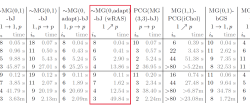Main results: multilevel a posteriori estimator of the algebraic error; guaranteed (reliable) and efficient: , ; polynomial-degree robust: β independent of p; gives rise to a multilevel iterative algebraic solver with contraction independent of the polynomial degree p: ; equivalence estimator – solver: α = √ (1 – β2); adaptive…

## Local- and global-best equivalence, simple projector, and optimal hp approximation in H(div)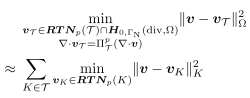Main results: global-best approximation (constraints on normal component continuity and divergence) is equivalent to the sum of independent local-best approximations (no constraints); gives rise to a simple stable local commuting projector in H(div); this projector delivers approximation error equivalent to the local-best approximation; leads to optimal hp approximation estimates in…

## Adaptive inexact semismooth Newton method for variational inequalities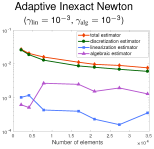Main results: guaranteed upper bound on the total error; distinction of different error components (spatial discretization, temporal discretization, semismooth Newton linearization, algebraic solver); design of an adaptive inexact semismooth Newton method for variational inequalities; application to unsteady nonlinear coupled degenerate variational inequalities (compositional two-phase flow in porous media with exchange…

## Goal-oriented adaptivity with inexact solvers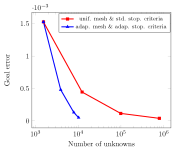Main results: quantity of interest given by a volumetric (source) or a surface (flux) term; same discretization of both primal and dual problems; inexact algebraic solvers for both primal and dual problems; guaranteed upper bound on the goal error; unified framework covering basically any numerical method (conforming, nonconforming, and discontinuous…

## Polytopal meshes and complex porous media flows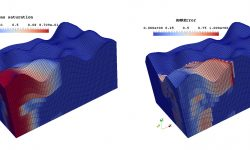Main results: general polytopal meshes; easy-to-implement and fast-to-run a posteriori error estimates given by a simple matrix–vector multiplication; works for any lowest-order locally conservative method; guaranteed upper bound on the total error; distinction of different error components (spatial discretization, temporal discretization, linearization, algebraic solver); unsteady nonlinear coupled degenerate problems (multiphase…

## Guaranteed bounds on the error reduction and hp adaptivity with inexact solversMain results: computable guaranteed bound on the error reduction factor (ratio of the errors between two successive hp refinements); homogeneous Neumann local problems via mixed finite elements to obtain error upper bound; homogeneous Dirichlet local problems via conforming finite elements for hp decision; hp-adaptive strategy with numerically exponential convergence rates;…